# Difference between revisions of "Norm"

A mapping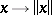from a vector spaceover the field of real or complex numbers into the real numbers, subject to the conditions: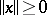, andfor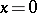only;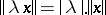for every scalar;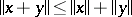for all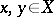(the triangle axiom). The number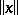is called the norm of the element.

A vector spacewith a distinguished norm is called a normed space. A norm induces ona metric by the formula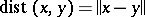, hence also a topology compatible with this metric. And so a normed space is endowed with the natural structure of a topological vector space. A normed space that is complete in this metric is called a Banach space. Every normed space has a Banach completion.

A topological vector space is said to be normable if its topology is compatible with some norm. Normability is equivalent to the existence of a convex bounded neighbourhood of zero (a theorem of Kolmogorov, 1934).

The norm in a normed vector spaceis generated by an inner product (that is,is isometrically isomorphic to a pre-Hilbert space) if and only if for all,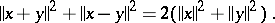Two norms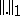and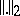on one and the same vector spaceare called equivalent if they induce the same topology. This comes to the same thing as the existence of two constants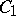and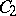such thatIfis complete in both norms, then their equivalence is a consequence of compatibility. Here compatibility means that the limit relationsimply that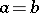.

Not every topological vector space, even if it is assumed to be locally convex, has a continuous norm. For example, there is no continuous norm on an infinite product of straight lines with the topology of coordinate-wise convergence. The absence of a continuous norm can be an obvious obstacle to the continuous imbedding of one topological vector space in another.

Ifis a closed subspace of a normed space, then the quotient space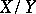of cosets bycan be endowed with the normunder which it becomes a normed space. The norm of the image of an elementunder the quotient mappingis called the quotient norm ofwith respect to.

The totality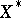of continuous linear functionalson a normed spaceforms a Banach space relative to the norm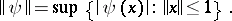The norms of all functionals are attained at suitable points of the unit ball of the original space if and only if the space is reflexive (cf. Reflexive space).

The totalityof continuous (bounded) linear operatorsfrom a normed spaceinto a normed spaceis made into a normed space by introducing the operator norm:Under this norm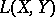is complete ifis. When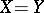is complete, the space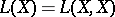with multiplication (composition) of operators becomes a Banach algebra, since for the operator norm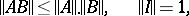whereis the identity operator (the unit element of the algebra). Other equivalent norms on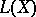subject to the same condition are also interesting. Such norms are sometimes called algebraic or ringed. Algebraic norms can be obtained by renormingequivalently and taking the corresponding operator norms; however, even fornot all algebraic norms oncan be obtained in this manner.

A pre-norm, or semi-norm, on a vector spaceis defined as a mappingwith the properties of a norm except non-degeneracy: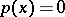does not preclude that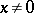. If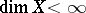, a non-zero pre-normonsubject to the condition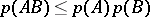actually turns out to be a norm (since in this casehas no non-trivial two-sided ideals). But for infinite-dimensional normed spaces this is not so. Ifis a Banach algebra over, then the spectral radius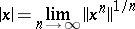is a semi-norm if and only if it is uniformly continuous on, and this condition is equivalent to the fact that the quotient algebra by the radical is commutative.